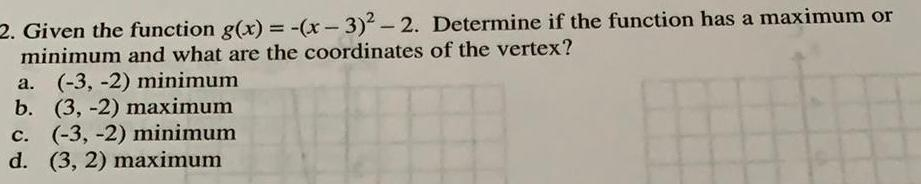Question:

# Given the function g(x) = -(x-3)² - 2. Determine if the

Last updated: 8/8/2022Given the function g(x) = -(x-3)² - 2. Determine if the function has a maximum or minimum and what are the coordinates of the vertex? a. (-3, -2) minimum b. (3,-2) maximum c. (-3, -2) minimum d. (3, 2) maximum